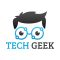Home » Core Java » Basic Operators – Java += OperatorThe author is graduated in Electronics & Telecommunication. During his studies, he has been involved with a significant number of projects ranging from programming and software engineering to telecommunications analysis. He works as a technical lead in the information technology sector where he is primarily involved with projects based on Java/J2EE technologies platform and novel UI technologies.

# Basic Operators – Java += Operator

Hello readers, in this tutorial, we will learn the Java += operator.

## 1. Introduction

Java programming offers Operators that are used to perform various operations in Java. In this tutorial, we’ll only cover the `+=` operator that falls under the Compound Assignment operators’ category.

### 1.1 Compound assignment operators in Java

Following table list the different compound assignment operators.

 Operator Description Syntax Is equivalent to? `+=` Compound addition assignment operator `ele2 += ele1` `ele2 = ele2+ele1` `-=` Compound subtraction assignment operator `ele2 -= ele1` `ele2 = ele2-ele1` `*=` Compound multiplication assignment operator `ele2 *= ele1` `ele2 = ele2*ele1` `/=` Compound division assignment operator `ele2 /= ele1` `ele2 = ele2/ele1` `%=` Compound modulo assignment operator `ele2 %= ele1` `ele2 = ele2%ele1`

To start with the captioned tutorial, we are hoping that users at present have their preferred Ide installed on their machines. For easy usage, I am using Eclipse Ide on a Windows operating system.

## 2. Basic Operators – Java += Operator

In this example, we’ll demonstrate the `+=` operator in java. For a better understanding, developers can execute the below code in Eclipse Ide.

Example.java

 0102030405060708091011121314151617181920 `package` `com.example;``public` `class` `Example {``    ``// Basic operators - Java += Operator.``    ``public` `static` `void` `main(String[] args) {``        ``int` `ele1 = ``5``;``        ``int` `ele2 = ``5``;``        ``ele2 += ele1;   ``// ele2 += ele1 is equal to ele2 = ele2+ele1;``        ``System.out.println(``"+= Output is: "``+ ele2);``String str1 = ``"Java"``;``        ``String str2 = ``"Geek"``;``        ``str2 += str1;   ``// str2 += str1 is equal to str2 = str2+str1;``        ``System.out.println(``"+= Output is: "``+ str2);``    ``}``}`

If everything goes well, the following output will be printed in the Ide console.

Output

 123 `+= Output: 10``+= Output: GeekJava`

That is all for this tutorial and I hope the article served you whatever you were looking for. Happy Learning and do not forget to share!

## 3. Java += Operator – Summary

In this tutorial, we learned the `+=` operator in Java. Developers can download the sample application as an Eclipse project in the Downloads section.

This was an example of += operator in Java programming language.

You can download the full source code of this example here: Basic Operators – Java += Operator(No Ratings Yet)
Start the discussion Views Tweet it!

# Do you want to know how to develop your skillset to become a Java Rockstar?

## Subscribe to our newsletter to start Rocking right now!

### and many more ....